Test Description

## 10 Questions MCQ Test | Test: Addition - 2

Test: Addition - 2 for Class 3 2023 is part of Class 3 preparation. The Test: Addition - 2 questions and answers have been prepared according to the Class 3 exam syllabus.The Test: Addition - 2 MCQs are made for Class 3 2023 Exam. Find important definitions, questions, notes, meanings, examples, exercises, MCQs and online tests for Test: Addition - 2 below.
Solutions of Test: Addition - 2 questions in English are available as part of our course for Class 3 & Test: Addition - 2 solutions in Hindi for Class 3 course. Download more important topics, notes, lectures and mock test series for Class 3 Exam by signing up for free. Attempt Test: Addition - 2 | 10 questions in 20 minutes | Mock test for Class 3 preparation | Free important questions MCQ to study for Class 3 Exam | Download free PDF with solutions
 1 Crore+ students have signed up on EduRev. Have you?
Test: Addition - 2 - Question 1

### Which number makes the equation true? 20 + 30 = 15 + ?

Detailed Solution for Test: Addition - 2 - Question 1

20+30=15+35=50

Test: Addition - 2 - Question 2

### Match the following: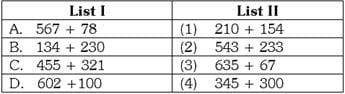Detailed Solution for Test: Addition - 2 - Question 2

567 + 78 = 645 = 345 + 300

134 + 230 = 364 = 210 + 154

455 + 321 = 776 = 543 + 233

602 + 100= 702 = 635 + 67

Test: Addition - 2 - Question 3

### Match the following: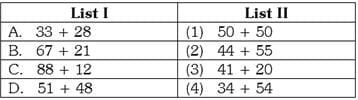Detailed Solution for Test: Addition - 2 - Question 3

33 + 28 = 61 = 41 + 20 67 + 21 = 88 = 34 + 54 88 + 12 = 100 = 50 + 50 51 + 48 = 99 = 44 + 55

Test: Addition - 2 - Question 4

The Venn diagram below shows the number of students who voted for their favorite sport. How many students voted in all?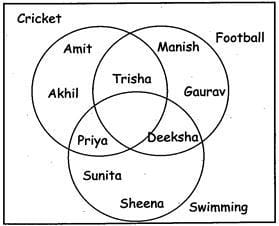Detailed Solution for Test: Addition - 2 - Question 4

Count the names of students in the diagram to calculate the total number of students who voted.

Test: Addition - 2 - Question 5

The diagram below shows how class III students voted for their favorite pet How many total number of students want dog or fish as their pet? Favorite Pet of Class III students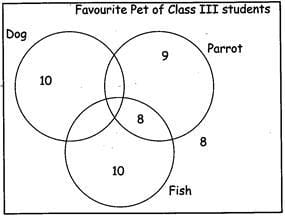Detailed Solution for Test: Addition - 2 - Question 5

Number of students who want dog as their pet = 10 Number of students who want Fish as their pet = 10+ 8 = 18 10 + 18 = 28.

Test: Addition - 2 - Question 6

Which numbers complete the pattern? 4 + 5= ___ 40 + 50 = ___ 400 + 500 = ____ 4000 + 5000 = ____

Detailed Solution for Test: Addition - 2 - Question 6

4+5 = 9 40+50 = 90 400 + 500 = 900 4000 + 5000 = 9000

Test: Addition - 2 - Question 7

Which numbers complete the pattern? ___ +6=8 ___ + 60= 80 ___ + 600= 800

Detailed Solution for Test: Addition - 2 - Question 7

8 ? 6 = 2 80 ? 60 = 20 800 ? 600 = 200

Test: Addition - 2 - Question 8

Look at the table below. What is rule for the table?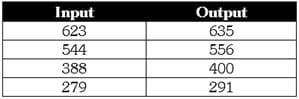Detailed Solution for Test: Addition - 2 - Question 8

623 + 12 = 635 544 + 12 = 556 388 + 12 = 400 279 + 12 = 291

Test: Addition - 2 - Question 9

Look at the table below. What is rule for the table?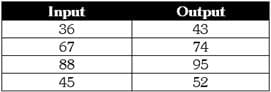Detailed Solution for Test: Addition - 2 - Question 9

36 + 7 = 43 67 + 7 =74 88 + 7 = 95 45 + 7 = 52

Test: Addition - 2 - Question 10

Fill in the table below. The rule is to Add 40 to each term.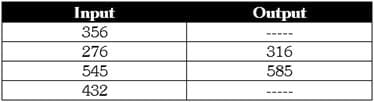Detailed Solution for Test: Addition - 2 - Question 10

356 + 40 = 396
432 + 40 = 472

 Use Code STAYHOME200 and get INR 200 additional OFF Use Coupon Code